## PREPARATORY QUESTIONS

### DATA INTERPRETATION (TABLE)

#### PREPARATORY SET-86 (TABLE)

Direction (Qs.1 to 7): Study the table below and answer the given questions.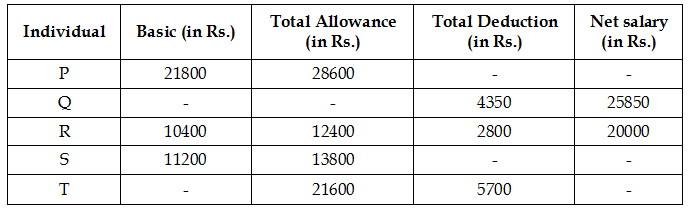Note:

(I) Total deduction = Provident Fund Deduction (which is 10% of basic salary) + Other deductions

(II) Net Salary = Basic Salary + Total Allowance – Total Deduction

(III)A few values are missing in the table (indicated by). A candidate is expected to calculate the missing value, if it is required to answer the given question on the basis of the given data and information

Question No : 1

If other deductions of P was Rs.4720, what was his net salary?

(1) Rs.42500

(2) Rs.43500

(3) Rs.43000

(4) Rs.41500

(5) Rs.42000

Question No : 2

If Q’s total allowance was Rs.3000 more than his basic salary, what was his total allowance?

(1) Rs.17000

(2) Rs.17500

(3) Rs.16000

(4) Rs.16600

(5) Rs.15500

Question No : 3

If the ratio of Provident Fund Deduction and Other deductions of S was 7 : 13, what was S’s Other deductions?

(1) Rs.2160

(2) Rs.2080

(3) Rs.2120

(4) Rs.2040

(5) Rs.1980

Question No : 4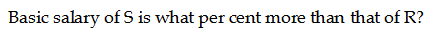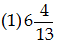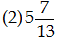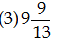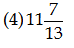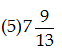Question No : 5

If other deduction of T was 4000 what was his net salary?

(1) Rs.32500

(2) Rs.31900

(3) Rs.32700

(4) Rs.31700

(5) Rs.32900

Question No : 6

If the ratio of the Provident fund and Other detection is 3 : 2,  If the total deduction is 10% of the basic salary of P then find the amount of other deduction?

(1) Rs.872

(2) Rs.972

(3) Rs.850

(4) Rs.829

(5) None of these

Question No : 7

Total allowance of P is what percent more than the total allowance of T?

(1) 38.20

(2) 25.95

(3) 32.40

(4) 29.2

(5) None of these